# Exploring GE14 results with Python (part 2)

See here for part 1.

# Motivation

DataTarik used feature importances from random forests to conclude that

• The ‘most important’ ethnic composition appears to be Chinese
• Ethnicity as a whole is related to election outcomes.

I argue here that demography considered jointly has a greater relationship with election outcomes.

# Introduction

Here are the feature variables expressed as a correlation matrix and visualised in a heatmap.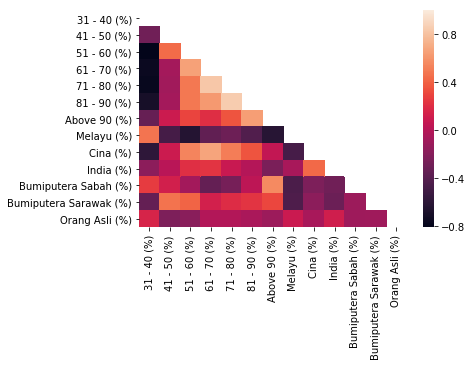Using the feature_importances implementation in scikit-learn, it’s unclear what’s going on when each feature tends to have strong associations with other features. Specifically, one feature tends to be the complement of another. If the algorithm tells that the proportion of Chinese is more important, perhaps the proportion of Chinese is inversely related with the proportion of Malays. If that is so, how do we tell which feature matters? Trying to interpret each feature as it is would be futile.

As discussed in explained.ai’s blog post (Beware Default Random Forest Importances), the default implementation of feature importance in scikit-learn is biased and susceptible to collinearity. More importantly, the features are almost perfectly collinear within each ‘meta-group’ of features. Many of the age and race variables sum to 100%.

Here is a plot of the distribution of the sum of age and race variables in the entire dataset.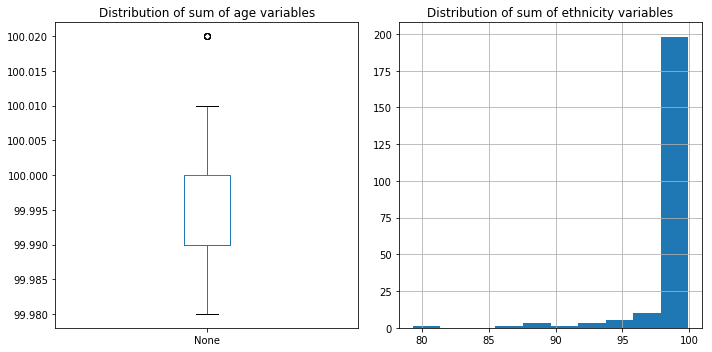To deal with the collinearity, Terence Parr, Kerem Turgutlu, Christopher Csiszar, and Jeremy Howard suggest to use drop-column importance in conjunction with the permutation importances metric. In contrast with the default feature importance metric, which measures the mean decrease in impurity, the permutation score permutates a column and then calculates the drop in the score from the baseline score. Do the following:

• compute baseline feature importance with permutation importance
• drop a column, retrain, and then recompute feature importance scores
• The importance score for a column is the difference between the baseline and the score for the model missing that column

### Drop-column

The feature importances from drop-column importances are shown as follows. These are computed using Terence Parr and Kerem Turgutlu’s rfpimp package: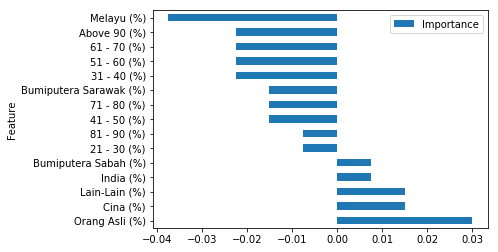Now, race matters but age doesn’t matter. Age has a negative permutation score - apparently permuting the age columns improves the model’s performance?

### Meta-features

A meta-features approach using permutation importance is another approach that can be taken here. rfpimp also provides an implementation of drop-column importances. The results are shown as follows: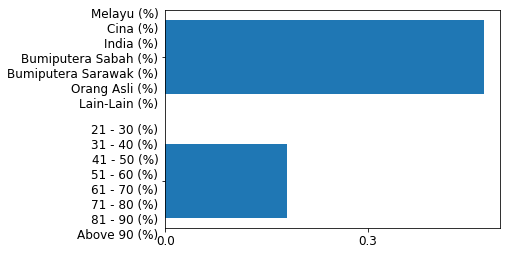Over 10 runs, the ratio of feature importance of the race- to age-meta-feature was:

[3.2307692307692304,
2.4666666666666672,
2.294117647058823,
3.2307692307692304,
2.058823529411764,
1.9999999999999996,
2.117647058823529,
3.3333333333333335,
2.8000000000000007,
2.235294117647059]


This confirms our result from drop-column that race matters here, but age doesn’t not matter.

But what if I consider all of them jointly? The permutation score skyrockets:and the permutation score over 10 runs is

[0.3932584269662921,
0.38202247191011235,
0.4044943820224719,
0.3033707865168539,
0.3595505617977528,
0.2134831460674157,
0.348314606741573,
0.3146067415730337,
0.2584269662921348,
0.348314606741573]


which is far higher than the permutation scores from age- and race-meta-features.

## Decision trees

To get a more interpretable model, we can use a decision tree model to fit the data. Using scikit-learn’s implementation of DecisionTreeClassifier, I get 71.2% accuracy on a test set of 40%. The decision tree can be found here. However, I don’t think it’s useful to answer our question either - it’s quite a complex tree, and it can’t give us a straight answer about whether age or race - or which aspects of age and race - are more important.

## PCA and demographic patterns

There is a potential use case for principal component analysis (PCA) here. Perhaps PCA can identify demographic patterns among the age and race variables. The figure below shows the ethnic breakdown from an inverse transformation of the first principal component. This is done by first transforming the ethnic variables by scikit-learn’s PCA implementation, then using scikit-learn’s inverse_transform function to transform the data back to its original space. This gives us an idea of what the first principal component is picking up in the data.

I haven’t investigated the data exhaustively, so I’ve picked 5 random numbers within the range of the first principal component and sorted them to have some sense of representativeness. It looks like the first principal component captures a spectrum of constituency groups from high-Chinese constituencies to high-Sabahan constituencies. Note that the first principal component only captures 28% of the variance.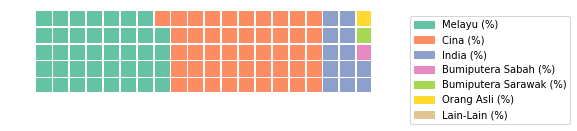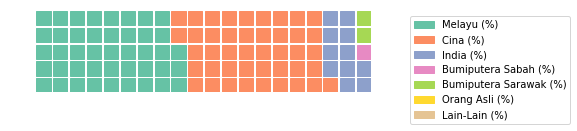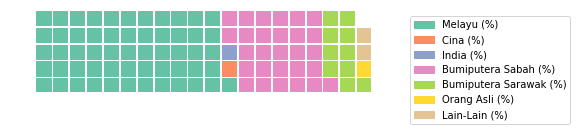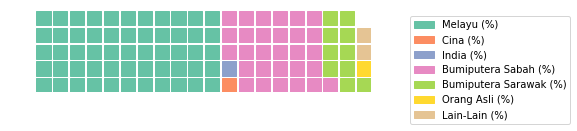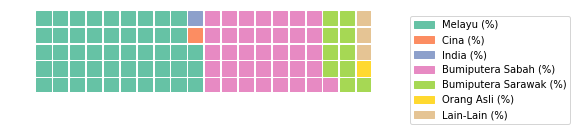The same exercise is done for the age variables. Its first principal component captures 61.4% of the variance. For age, PCA has an easier job - it just sorts between constituencies with left-skewed and right-skewed age distributions.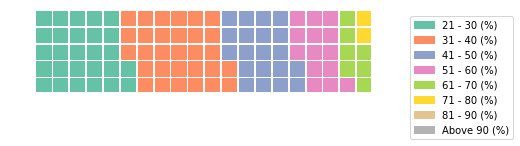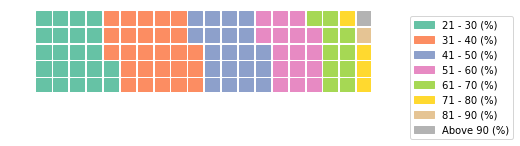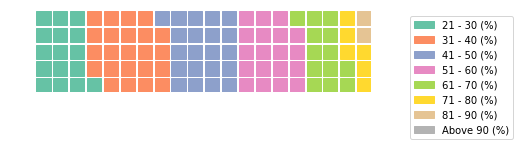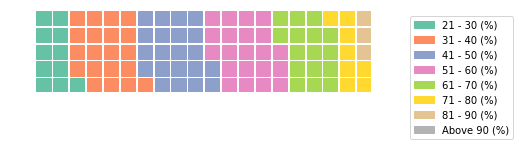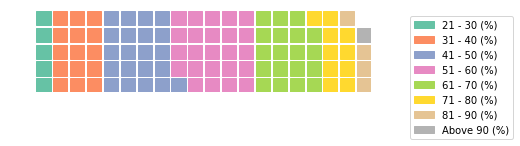## Multinomial logit + PCA

We can see how these patterns identified via PCA affect electoral outcomes using a multinomial logit model. A multinomial logit allows us to obtain relative and marginal probabilities of each class, so that we can interpret the model for each contesting party. Note that PCA is not the best approach for this. Lubostky and Wittenberg (2002) note that it is not clear that the PCA procedure helps with capturing the structural relationship between a latent variable and an outcome of interest.

(I estimated my multinomial logit on a 70% split of the data. This is an arbitrary decision, but since prediction is not the core task of this model, I’m not too interested in evaluating its predictive power.)

Using statsmodels’s MNLogit, the regression statistics are as follows. This model predicts with 16% accuracy out-of-sample.

Dep. Variable: No. Observations: y 155 MNLogit 147 MLE 4 Sun, 26 Aug 2018 -0.2011 15:34:15 -203.94 True -169.8 1
y=BN coef std err z P>|z| [0.025 0.975]
Race 0.3883 0.211 1.838 0.066 -0.026 0.802
Age -0.3440 0.151 -2.275 0.023 -0.640 -0.048
y=Gagasan Sejahtera coef std err z P>|z| [0.025 0.975]
Race 0.0476 0.240 0.198 0.843 -0.424 0.519
Age -0.3428 0.154 -2.222 0.026 -0.645 -0.040
y=PH coef std err z P>|z| [0.025 0.975]
Race -1.4327 0.275 -5.216 0.000 -1.971 -0.894
Age 0.3323 0.150 2.216 0.027 0.038 0.626
y=WARISAN coef std err z P>|z| [0.025 0.975]
Race 0.3364 0.215 1.568 0.117 -0.084 0.757
Age -0.2115 0.153 -1.380 0.167 -0.512 0.089

### Relative odds

The coefficients are expressed in log-odds, so these are exponentiated to obtain relative odds. The relative odds can be interpreted as the probability relative to BEBAS (independent candidate) being improved by the relative odds if Race/Age increased by one standard deviation.

BN Gagasan Sejahtera PH WARISAN
Race 1.474485 1.048732 0.238672 1.399909
Age 0.708961 0.709755 1.394134 0.809366

### Marginal odds

We can tell a slightly different story with marginal odds. The advantage of using marginal odds is that we can get an idea of actual, rather than relative probabilities (see here for details), however they can be quite tricky to interpret. The marginal effect for a continuous variable $X_k$ provides the instantaneous rate of change if the variable $X_k$ increased by an infinitesimal amount $\Delta$, holding all other variables $X$ constant. See this for details. $$\lim_{\Delta \to 0} \Pr(Y = 1|X, X_k+\Delta)- \Pr(y=1|X, X_k)$$

In other words, what is the change in (log-)probabiity if the first principal component changes by an infinitesimal amount?

The means of each principal component will coincide with 0, therefore at 0 I get marginal effects at average race/age. This is visualised below.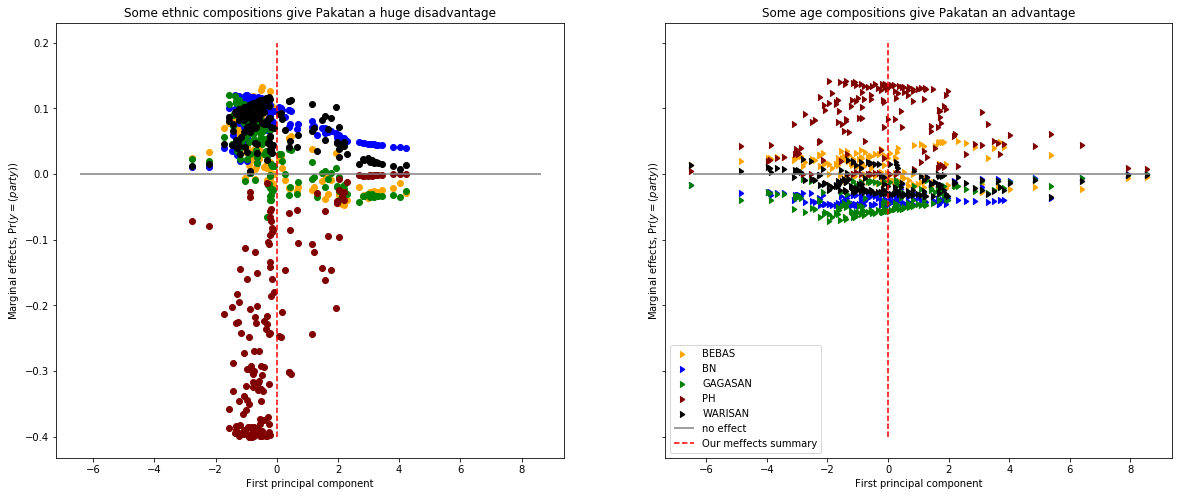# Lubotsky-Wittenberg post-hoc estimator for multiple proxies

Lubotsky and Wittenberg (2002) proposed a method to interpret the coefficients in a regression under the null hypothesis that the variables are all generated by a common latent factor. Let the latent factor $x_{i}^*$ be measured by $j$ proxy variables $z_{ji}$, where each $z_{ji}$ is related to the latent factor by $$z_{ji}=\rho x^*_i + \mu _{ij}$$

The goal is to measure the effect of $x^*$ on $y$: $$y_i = \beta_{LW} x^*_{it}+\epsilon_i$$

I follow the estimation procedure in Vosters & Nybom (2016):

1. Fit the regression model: $$y_i=\phi_1 z_{1i} + \phi_2 z_{2i} + ...$$

2. Estimate $\rho_j$ of each $z_j$ (except $z_1$, where $\rho_j$ is normalised to 1) using two-stage least squares. The procedure is akin to instrumental variables: use $z_j$ as outcome and $y$ as instrument for $z_1$.

3. Calculate $$\beta_{LW}=\rho_1\phi_1 + \rho_2 \phi_2 + \rho_3 \phi_3 + ... + \rho_k \phi_k$$ where $\rho_1 = 1$.

To be on the safe side, I assume a linear probability model, where the outcome of interest is whether Pakatan Harapan wins the seat. I’m not too familiar with the literature, but I couldn’t really find it being used in a classification problem before, so I’m not sure if it’s shown to be appropriate for the logistic model.

And since I can’t figure out how to properly construct my standard errors, you’ll just have to make do with estimates.

The F-stat is provided for the first stage: a rule of thumb is to have an F-stat which exceeds 10. The F-stat tests whether the instrument is ‘relevant’.

• I write two functions: lw and lw_controls: one function provides the L-W estimate without controls, and the other with controls.
• My training set is similar to the previous training set for the multinomial logit.
• I start out estimating without controls - this gives me extremely small effects. If Race is independent of Age (e.g. diagram below), the estimation is unbiased.
Race Age
F-stat 65.430 119.093
$\hat \beta_{LW}$ .00073 -.00053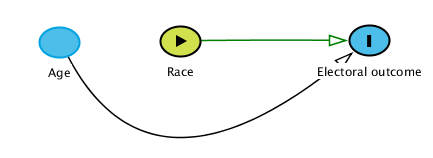• Estimating the effect of ‘race’ conditional on age (and vice versa) gives me larger effects. This suggests that there’s attenuation bias from omitting controls. These are ‘good’ estimates if race confounds age, or age confounds race.
Race Age
F-stat 32.288 96.985
$\hat \beta_{LW}$ -0.041 0.072
• However, it’s not clear that demographic factors should be considered separately. Putting race and ethnicity variables together, the estimated L-W effect spikes.
Demography
F-stat 119.093
$\hat \beta_{LW}$ 0.971

Interestingly, this result seems to confirm what we’ve seen in the random forests feature importances scores.

This estimate is OK assuming there are no confounders for the relationship between demography and electoral outcomes. For example, demography could be confounded by economic conditions that affect both the demographies of the region and preferences of the electorate.

# Conclusions

Interpreting feature importance metrics should be done with caution, particularly with random forests. The results suggest that there is interaction within and between age and ethnicity variables that contributes to electoral performance. The way I think of it, there are age-race combinations that are important contributors to electoral performance. Random Forests help with detecting interactions, however it’s not clear how these can be disentangled in feature importances metrics.

Also, the effect of demography could be reflective of unmeasured confounders. Without carefully considering the determinants of electoral performance, it’s not clear if demography is of such critical importance to electoral outcomes.

Notes:

1. I started out being critical with the 10% test set (23 observations). But the author of the original article set out to do estimation and not prediction, so I don’t think it’s too big a deal. In any case, I used test sets between 30 and 40% for this article.
2. The data is scaled before it is transformed by PCA, and the data is inverse-transformed by the original scaler before constructing these charts. Variables with negative inverse-transformed values are set to zero.